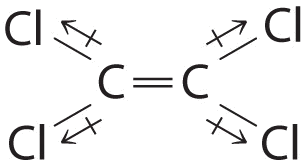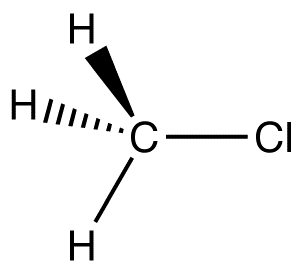8.3: The Reversibility of an $$S_N2$$ Reaction Depends on the Basicities of the Leaving Groups in the Forward and Reverse Directions

Dipole moments occur when there is a separation of charge. They can occur between two ions in an ionic bond or between atoms in a covalent bond; dipole moments arise from differences in electronegativity. The larger the difference in electronegativity, the larger the dipole moment. The distance between the charge separation is also a deciding factor into the size of the dipole moment. The dipole moment is a measure of the polarity of the molecule.

Introduction

When atoms in a molecule share electrons unequally, they create what is called a dipole moment. This occurs when one atom is more electronegative than another, resulting in that atom pulling more tightly on the shared pair of electrons, or when one atom has a lone pair of electrons and the difference of electronegativity vector points in the same way. One of the most common examples is the water molecule, made up of one oxygen atom and two hydrogen atoms. The differences in electronegativity and lone electrons give oxygen a partial negative charge and each hydrogen a partial positive charge.

Dipole Moment

When two electrical charges, of opposite sign and equal magnitude, are separated by a distance, an electric dipole is established. The size of a dipole is measured by its dipole moment ($$\mu$$). Dipole moment is measured in Debye units, which is equal to the distance between the charges multiplied by the charge (1 Debye equals $$3.34 \times 10^{-30}\; C\, m$$). The dipole moment of a molecule can be calculated by Equation $$\ref{1}$$:

$\vec{\mu} = \sum_i q_i \, \vec{r}_i \label{1}$

where

• $$\vec{\mu}$$ is the dipole moment vector
• $$q_i$$ is the magnitude of the $$i^{th}$$ charge, and
• $$\vec{r}_i$$ is the vector representing the position of $$i^{th}$$ charge.

The dipole moment acts in the direction of the vector quantity. An example of a polar molecule is $$\ce{H_2O}$$. Because of the lone pair on oxygen, the structure of $$\ce{H_2O}$$ is bent (via VEPSR theory), which that the vectors representing the dipole moment of each bond do not cancel each other out. Hence, water is polar.Figure $$\PageIndex{1}$$​: Dipole moment of water. The convention in chemistry is that the arrow representing the dipole moment goes from positive to negative. Physicist tend to use the opposite orientation.

The vector points from positive to negative, on both the molecular (net) dipole moment and the individual bond dipoles. Table A2 shows the electronegativity of some of the common elements. The larger the difference in electronegativity between the two atoms, the more electronegative that bond is. To be considered a polar bond, the difference in electronegativity must be large. The dipole moment points in the direction of the vector quantity of each of the bond electronegativities added together.

It is relatively easy to measure dipole moments; just place a substance between charged plates (Figure $$\PageIndex{2}$$) and polar molecules increase the charge stored on plates and the dipole moment can be obtained (i.e., via the capacitance of the system). ​Nonpolar $$\ce{CCl_4}$$ is not deflected; moderately polar acetone deflects slightly; highly polar water deflects strongly. In general, polar molecules will align themselves: (1) in an electric field, (2) with respect to one another, or (3) with respect to ions (Figure $$\PageIndex{2}$$).Figure $$\PageIndex{2}$$: Polar molecules align themselves in an electric field (left), with respect to one another (middle), and with respect to ions (right)

Equation $$\ref{1}$$ can be simplified for a simple separated two-charge system like diatomic molecules or when considering a bond dipole within a molecule

$\mu_{diatomic} = Q \times r \label{1a}$

This bond dipole is interpreted as the dipole from a charge separation over a distance $$r$$ between the partial charges $$Q^+$$ and $$Q^-$$ (or the more commonly used terms $$δ^+$$ - $$δ^-$$); the orientation of the dipole is along the axis of the bond. Consider a simple system of a single electron and proton separated by a fix distance. When proton and electron close together, the dipole moment (degree of polarity) decreases. However, as proton and electron get farther apart, the dipole moment increases. In this case, the dipole moment calculated as (via Equation $$\ref{1a}$$):

\begin{align} \mu &= Qr \nonumber \\[4pt] &= (1.60 \times 10^{-19}\, C)(1.00 \times 10^{-10} \,m) \nonumber \\[4pt] &= 1.60 \times 10^{-29} \,C \cdot m \label{2} \end{align}

The Debye characterizes size of dipole moment. When a proton & electron 100 pm apart, the dipole moment is $$4.80\; D$$:

\begin{align} \mu &= (1.60 \times 10^{-29}\, C \cdot m) \left(\dfrac{1 \;D}{3.336 \times 10^{-30} \, C \cdot m} \right) \nonumber \\[4pt] &= 4.80\; D \label{3} \end{align}

$$4.80\; D$$ is a key reference value and represents a pure charge of +1 and -1 separated by 100 pm. If the charge separation were increased then the dipole moment increases (linearly):

• If the proton and electron were separated by 120 pm:

$\mu = \dfrac{120}{100}(4.80\;D) = 5.76\, D \label{4a}$

• If the proton and electron were separated by 150 pm:

$\mu = \dfrac{150}{100}(4.80 \; D) = 7.20\, D \label{4b}$

• If the proton and electron were separated by 200 pm:

$\mu = \dfrac{200}{100}(4.80 \; D) = 9.60 \,D \label{4c}$

Example $$\PageIndex{1}$$: Water

The water molecule in Figure $$\PageIndex{1}$$ can be used to determine the direction and magnitude of the dipole moment. From the electronegativities of oxygen and hydrogen, the difference is 1.2e for each of the hydrogen-oxygen bonds. Next, because the oxygen is the more electronegative atom, it exerts a greater pull on the shared electrons; it also has two lone pairs of electrons. From this, it can be concluded that the dipole moment points from between the two hydrogen atoms toward the oxygen atom. Using the equation above, the dipole moment is calculated to be 1.85 D by multiplying the distance between the oxygen and hydrogen atoms by the charge difference between them and then finding the components of each that point in the direction of the net dipole moment (the angle of the molecule is 104.5˚).

The bond moment of O-H bond =1.5 D, so the net dipole moment is

$\mu=2(1.5) \cos \left(\dfrac{104.5˚}{2}\right)=1.84\; D \nonumber$

Polarity and Structure of Molecules

The shape of a molecule and the polarity of its bonds determine the OVERALL POLARITY of that molecule. A molecule that contains polar bonds, might not have any overall polarity, depending upon its shape. The simple definition of whether a complex molecule is polar or not depends upon whether its overall centers of positive and negative charges overlap. If these centers lie at the same point in space, then the molecule has no overall polarity (and is non polar). If a molecule is completely symmetric, then the dipole moment vectors on each molecule will cancel each other out, making the molecule nonpolar. A molecule can only be polar if the structure of that molecule is not symmetric.Figure $$\PageIndex{3}$$: Charge distributions of $$CO_2$$ and $$H_2O$$. Blue and red colored regions are negative and positively signed regions, respectively.

A good example of a nonpolar molecule that contains polar bonds is carbon dioxide (Figure $$\PageIndex{3a}$$). This is a linear molecule and each C=O bond is, in fact, polar. The central carbon will have a net positive charge, and the two outer oxygen atoms a net negative charge. However, since the molecule is linear, these two bond dipoles cancel each other out (i.e. the vector addition of the dipoles equals zero) and the overall molecule has a zero dipole moment ($$\mu=0$$).

Although a polar bond is a prerequisite for a molecule to have a dipole, not all molecules with polar bonds exhibit dipoles

For $$AB_n$$ molecules, where $$A$$ is the central atom and $$B$$ are all the same types of atoms, there are certain molecular geometries which are symmetric. Therefore, they will have no dipole even if the bonds are polar. These geometries include linear, trigonal planar, tetrahedral, octahedral and trigonal ​bipyramid.Figure $$\PageIndex{4}$$: Molecular geometries with exact cancellation of polar bonding to generate a non-polar molecule ($$\mu=0$$)

Example $$\PageIndex{3}$$: $$\ce{C_2Cl_4}$$

Although the C–Cl bonds are rather polar, the individual bond dipoles cancel one another in this symmetrical structure, and $$\ce{Cl_2C=CCl_2}$$ does not have a net dipole moment.Example $$\PageIndex{3}$$: $$\ce{CH_3Cl}$$

C-Cl, the key polar bond, is 178 pm. Measurement reveals 1.87 D. From this data, % ionic character can be computed. If this bond were 100% ionic (based on proton & electron),

\begin{align} \mu &= \dfrac{178}{100}(4.80\; D) \nonumber \\[4pt] &= 8.54\; D \nonumber \end{align} \nonumberAlthough the bond length is increasing, the dipole is decreasing as you move down the halogen group. The electronegativity decreases as we move down the group. Thus, the greater influence is the electronegativity of the two atoms (which influences the charge at the ends of the dipole).

Table $$\PageIndex{1}$$: Relationship between Bond length, Electronegativity and Dipole moments in simple Diatomics
Compound Bond Length (Å) Electronegativity Difference Dipole Moment (D)
HF 0.92 1.9 1.82
HCl 1.27 0.9 1.08
HBr 1.41 0.7 0.82
HI 1.61 0.4 0.44

References

1. Housecroft, Catherine E. and Alan G. Sharpe. Inorganic Chemistry. 3rd ed. Harlow: Pearson Education, 2008. Print. (Pages 44-46)
2. Tro, Nivaldo J. Chemistry: A Molecular Approach. Upper Saddle River: Pearson Education, 2008. Print. (Pages 379-386)